# Perimeter Of Shapes

We come across many shapes in daily life.  Consider a triangle, a rectangle, a square or any other shape. Every shape begins with a line or a line segment or a curve. Number and arrangement of these lines decide the type of figure. Each shape has an interior region or surface bounded by these lines. These bounded lines form the perimeter of a geometric shape and formula for it may vary from one shape to another.

## What is the Perimeter?

Any shape that lies on a flat surface and has only two dimensions i.e. length and breadth are called 2-D (two-dimensional) shape. Every polygon is a 2-D figure which lies flat on a plane. Polygons are closed figures which are bounded by a chain of line segments, for example, triangles, rectangles, and squares. Perimeter (peri: around; meter: measure) of a polygon is the distance or linear measure of these bounded line segments. In other words, it is the length of its boundaries. Its unit is centimeter (cm) or meter (m).

Consider a rectangle, the surrounding distance indicated by the arrows form the perimeter of the given rectangle. It is the sum total covered by two lengths and two breadths.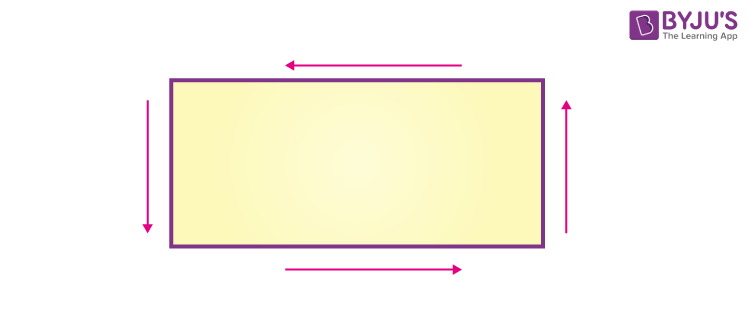## Perimeter of Shapes Formula

As mentioned above, perimeter of any shape can be calculated considering the boundary of the shape. Thus, the formula of perimeter of shapes is given by:

Perimeter of shapes = Total length of the boundary of shapes

## Perimeter of Different Shapes – Formulas

We know every shape is different from one another. Thus, the formula for perimeters of geometric shapes also varies from one shape to another. Some common shapes and formula for finding their perimeters are as follows.

### Perimeter of Rectangle

Rectangle is a four-sided polygon having two dimensions i.e. length and breadth.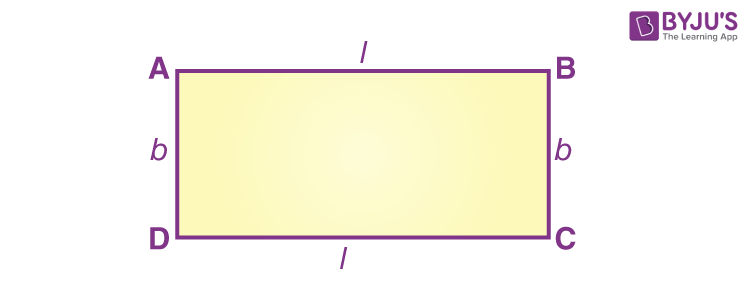Perimeter of a rectangle = sum of four sides

= AB     +     BC        + CD       +   AD

= 2 length + 2 breadth

Perimeter of a rectangle =   2 × (length + breadth)

Also, get the perimeter formulas for all geometrical shapes here.

### Perimeter of Circle

Circle is a collection of points and perimeter of a circle is known as circumference of a circle.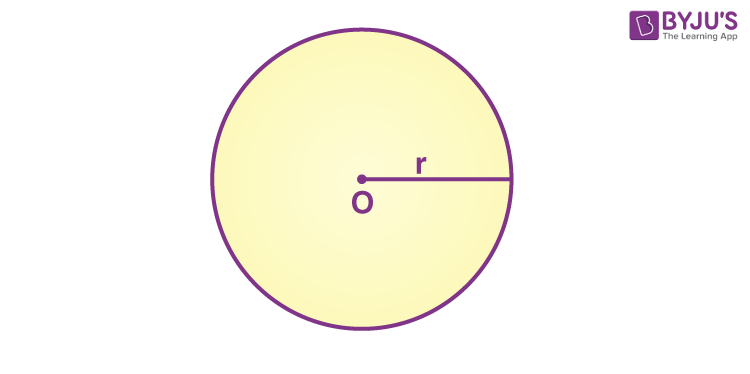Circumference of a circle is equal to 2πr.

Where r is the radius of the circle and

### Perimeter of Square

Square is also a polygon where all its sides are the same.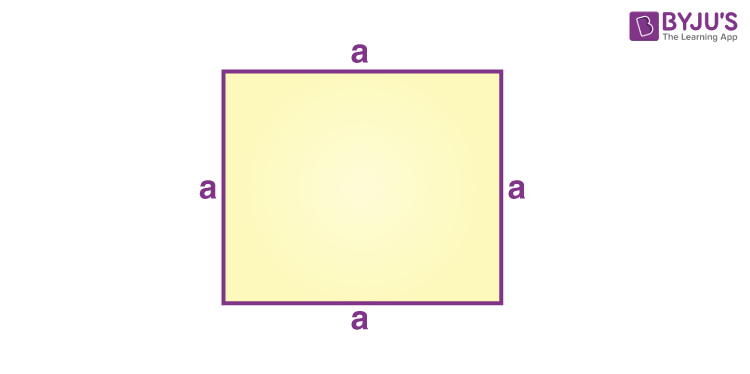The perimeter of a square = sum of all four sides

= a + a + a+ a

= 4a

The perimeter of a square = 4 × side

These are a few examples of perimeter of shapes that we use frequently. However, we can find perimeter for all the 2d shapes.

### Perimeter of Shapes Questions

Example 1: Find the perimeter of the given shape.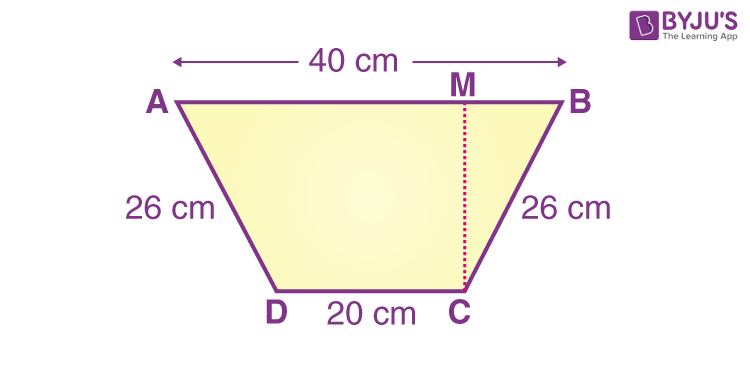Solution:

In the given figure,

AB = 40 cm

BC = 26 cm

CD = 20 cm

DA = 26 cm

Perimeter of the shape = AB + BC + CD + DA

= (40 + 26 + 20 + 26) cm

= 112 cm

Example 2: What is the perimeter of a square with side 9 cm?

Solution:

Given,

Length of the side of a square = a = 9 cm

Perimeter of the square = 4a

= 4 ×9 cm

= 36 cm

#### Watch The Below Video To Understand The Distance Around The Shapes